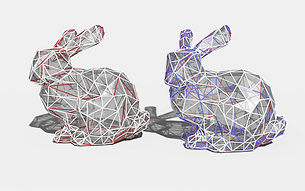top of page

## Summary

We are going to implement several parallel algorithms for the Delaunay triangulation in OpenMP and OpenMPI.## Background

Delaunay triangulation is an important problem in mathematics and computational geometry. Here we are interested in the 2-d version of the problem. Given a set of points in a plane, the Delaunay triangulation divides the plane into the exterior region (outside the convex hull) and the interior region, which consists of triangles such that the circumcircle of any triangle contains no other points. The resulting triangulation maximizes the minimum of all angles in the triangles. Visually, the Delaunay triangulation tends to avoid “thing” triangles.

The Delaunay triangulation has many interesting applications. Algorithmically, it is a superset of the minimum spanning tree of the same vertex set. It’s also the dual graph of the Voroni diagram, which is the partition of the space into regions that are closest to each vertex.

Practically, Delaunay triangulation in higher dimensions is especially useful for mesh generation and physical simulation.## Challenges

Algorithm design will be the key challenge of this project.
The sequential algorithm of Delaunay triangulation is non-trivial to begin with. Being a central topic in computational geometry, many algorithms rely on critical geometry primitives to implement and optimize. While the incremental insertion algorithm is easy to understand, it is inherently hard to parallelize.

A brief survey of related work gives us some insight into how parallelization might come into play here. First, it is possible to utilize a tradeoff between work-efficiency and scalability. Paper by Craig and Chen proposed one such solution, where by doing more “local” work for each vertex, it is possible to achieve almost linear speed up with multiprocessing. Also, a randomized incremental algorithm is proposed by Gu et al, where insertion of many new points are done concurrently, making it possible to parallelize each iteration of the algorithm.

General problems with parallel computation can be observed here as well. In terms of workload, since the insertion of each point is non-deterministic, in the sense that we don’t know how many “flips” it will trigger, it is important that we come up with some dynamic workload balancing mechanism. Locality is extremely important in the divide-and-conquer style algorithm, as well as in managing the frequent memory operations associated with keeping track of geometric properties such as vertices, edges, triangles, and etc.## Resources

As mentioned above, we would like to reference a lot of papers on both sequential and parallel implementations of Delaunay triangulation. A partial list of papers can be found at the end of this proposal.

In terms of starter code, we would like to start building our parallel implementation on top of some working version of sequential code. The exact choice is undecided yet, mainly depending on the parallel algorithm we choose later on. But either way, we would like to use a divide and conquer algorithm as a baseline to measure our performance, since it is shown to be the fastest sequential DT generation technique.

### Goals & Deliverables

###### Plan to achieve:
• A sequential version of Delaunay triangulation

• A parallel version of Delaunay triangulation extended from the

incremental insertion algorithm (possibly in openMP)

• A parallel version of Delaunay triangulation extended from the

divide and conquer algorithm (possibly in openMP)

###### Hope to achieve:
• A parallel version of Delaunay triangulation in openMPI

• Runtime decomposition and performance analysis compared to the other implementations

###### Fall back Plan:
• Finish one of the two parallel implementations described in plan to achieve

###### Deliverables:
• Speed up diagram of parallel implementations compared to the best sequential implementation

• Since the parallel algorithms might be non-trivial, we are planning to come up with some interactive output that demonstrates how they run on different input## Platform Choice

The incremental insertion algorithm has the property that each insertion has unpredictable workload, depending on the number of subsequent flips. Thus, a dynamic workload balancing mechanism seems to come in handy, which is why we are leaning towards OpenMP at this point.

The divide and conquer algorithm is a recursive algorithm that doesn’t work well with OpenMPI. We might explore Cilk later on as well.

## Schedule

1 / WEEK 1 (11/9 - 11/15)

Prepare for exams and study various sequential algorithms.

##### 3 / WEEK 3 (11/23 - 11/29)
1. Fix potential problems with sequential algorithms.

2. Finish first parallel implementation based on incremental insertion.

##### 5 / WEEK 5 (12/7 - 12/13)
1. Performance debugging for second parallel version

2. Performance analysis

2 / WEEK 2 (11/16 - 11/22)

Do literature review for possible parallel algorithms and choose appropriate starter code.

##### 4 / WEEK 4 (11/30 - 12/6)
1. Performance debugging for first parallel version

2. Finish second parallel implementation based on divide and conquer.

##### 6 / WEEK 6 (12/14 - 12-18)
1. Preparing visualization tool for final presentation

2. Preparing poster

## References

1. Wikipedia contributors. (2022, September 20). Delaunay triangulation. Wikipedia. https://en.wikipedia.org/wiki/Delaunay_triangulation
2. Chen, R., & Gotsman, C. (2013). Localizing the Delaunay triangulation and its parallel implementation. In Transactions on Computational Science XX (pp. 39-55). Springer, Berlin, Heidelberg.
3. Blelloch, G. E., Gu, Y., Shun, J., & Sun, Y. (2020). Parallelism in randomized incremental algorithms. Journal of the ACM (JACM), 67(5), 1-27.
4. Cignoni, P., Montani, C., & Scopigno, R. (1998). DeWall: A fast divide and conquer Delaunay triangulation algorithm in Ed. Computer-Aided Design, 30(5), 333-341.
5.Shewchuk, J. R. (1997). Delaunay refinement mesh generation. Carnegie Mellon University.

bottom of page